## Routing Algorithms-

• Routing algorithms are meant for determining the routing of packets in a node.
• Routing algorithms are classified as-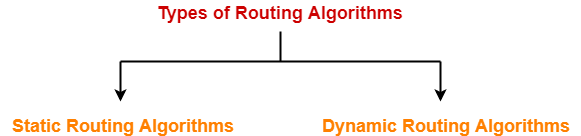1. Static Routing Algorithms
2. Dynamic Routing Algorithms

## Distance Vector Routing Algorithm-

 Distance Vector Routing is a dynamic routing algorithm.

It works in the following steps-

## Step-01:

Each router prepares its routing table. By their local knowledge. each router knows about-

• All the routers present in the network
• Distance to its neighboring routers

## Step-02:

• Each router exchanges its distance vector with its neighboring routers.
• Each router prepares a new routing table using the distance vectors it has obtained from its neighbors.
• This step is repeated for (n-2) times if there are n routers in the network.
• After this, routing tables converge / become stable.

## Distance Vector Routing Example-

Consider-

• There is a network consisting of 4 routers.
• The weights are mentioned on the edges.
• Weights could be distances or costs or delays.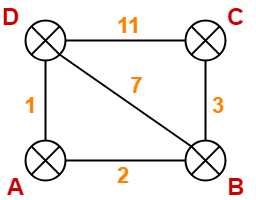## Step-01:

Each router prepares its routing table using its local knowledge.

Routing table prepared by each router is shown below-

### At Router A-

 Destination Distance Next Hop A 0 A B 2 B C ∞ – D 1 D

### At Router B-

 Destination Distance Next Hop A 2 A B 0 B C 3 C D 7 D

### At Router C-

 Destination Distance Next Hop A ∞ – B 3 B C 0 C D 11 D

### At Router D-

 Destination Distance Next Hop A 1 A B 7 B C 11 C D 0 D

## Step-02:

• Each router exchanges its distance vector obtained in Step-01 with its neighbors.
• After exchanging the distance vectors, each router prepares a new routing table.

This is shown below-

### At Router A-

• Router A receives distance vectors from its neighbors B and D.
• Router A prepares a new routing table as-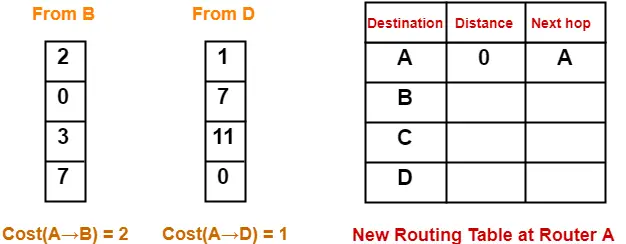• Cost of reaching destination B from router A = min { 2+0 , 1+7 } = 2 via B.
• Cost of reaching destination C from router A = min { 2+3 , 1+11 } = 5 via B.
• Cost of reaching destination D from router A = min { 2+7 , 1+0 } = 1 via D.

### Explanation For Destination B

• Router A can reach the destination router B via its neighbor B or neighbor D.
• It chooses the path which gives the minimum cost.
• Cost of reaching router B from router A via neighbor B = Cost (A→B) + Cost (B→B)= 2 + 0 = 2
• Cost of reaching router B from router A via neighbor D = Cost (A→D) + Cost (D→B) = 1 + 7 = 8
• Since the cost is minimum via neighbor B, so router A chooses the path via B.
• It creates an entry (2, B) for destination B in its new routing table.
• Similarly, we calculate the shortest path distance to each destination router at every router.

Thus, the new routing table at router A is-

 Destination Distance Next Hop A 0 A B 2 B C 5 B D 1 D

### At Router B-

• Router B receives distance vectors from its neighbors A, C and D.
• Router B prepares a new routing table as-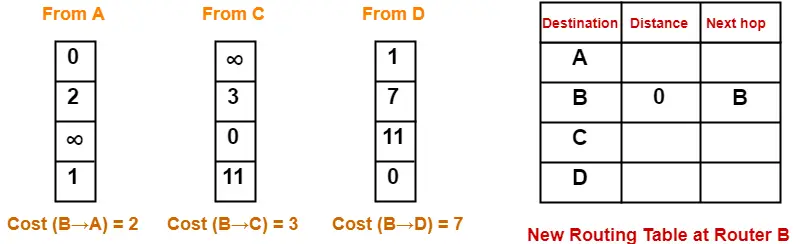• Cost of reaching destination A from router B = min { 2+0 , 3+∞ , 7+1 } = 2 via A.
• Cost of reaching destination C from router B = min { 2+∞ , 3+0 , 7+11 } = 3 via C.
• Cost of reaching destination D from router B = min { 2+1 , 3+11 , 7+0 } = 3 via A.

Thus, the new routing table at router B is-

 Destination Distance Next Hop A 2 A B 0 B C 3 C D 3 A

### At Router C-

• Router C receives distance vectors from its neighbors B and D.
• Router C prepares a new routing table as-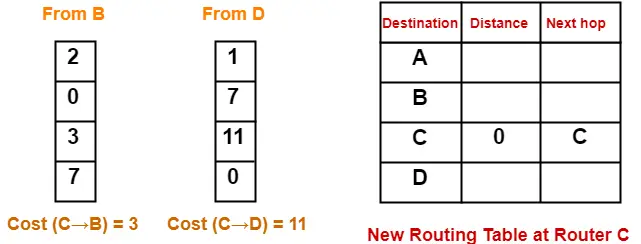• Cost of reaching destination A from router C = min { 3+2 , 11+1 } = 5 via B.
• Cost of reaching destination B from router C = min { 3+0 , 11+7 } = 3 via B.
• Cost of reaching destination D from router C = min { 3+7 , 11+0 } = 10 via B.

Thus, the new routing table at router C is-

 Destination Distance Next Hop A 5 B B 3 B C 0 C D 10 B

### At Router D-

• Router D receives distance vectors from its neighbors A, B and C.
• Router D prepares a new routing table as-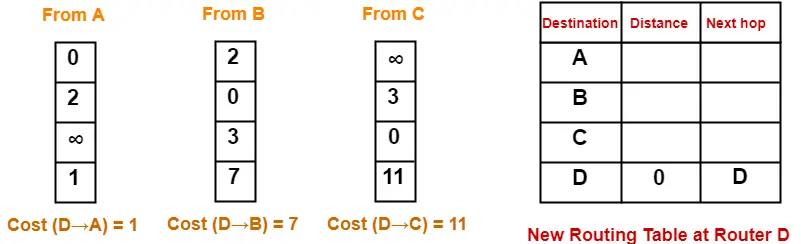• Cost of reaching destination A from router D = min { 1+0 , 7+2 , 11+∞ } = 1 via A.
• Cost of reaching destination B from router D = min { 1+2 , 7+0 , 11+3 } = 3 via A.
• Cost of reaching destination C from router D = min { 1+∞ , 7+3 , 11+0 } = 10 via B.

Thus, the new routing table at router D is-

 Destination Distance Next Hop A 1 A B 3 A C 10 B D 0 D

## Step-03:

• Each router exchanges its distance vector obtained in Step-02 with its neighboring routers.
• After exchanging the distance vectors, each router prepares a new routing table.

This is shown below-

### At Router A-

• Router A receives distance vectors from its neighbors B and D.
• Router A prepares a new routing table as-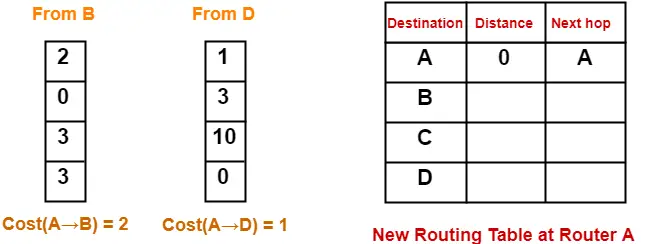• Cost of reaching destination B from router A = min { 2+0 , 1+3 } = 2 via B.
• Cost of reaching destination C from router A = min { 2+3 , 1+10 } = 5 via B.
• Cost of reaching destination D from router A = min { 2+3 , 1+0 } = 1 via D.

Thus, the new routing table at router A is-

 Destination Distance Next Hop A 0 A B 2 B C 5 B D 1 D

### At Router B-

• Router B receives distance vectors from its neighbors A, C and D.
• Router B prepares a new routing table as-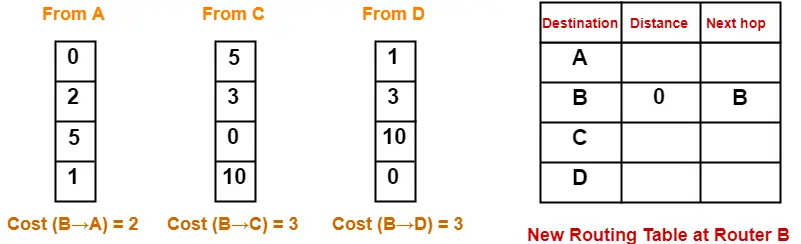• Cost of reaching destination A from router B = min { 2+0 , 3+5 , 3+1 } = 2 via A.
• Cost of reaching destination C from router B = min { 2+5 , 3+0 , 3+10 } = 3 via C.
• Cost of reaching destination D from router B = min { 2+1 , 3+10 , 3+0 } = 3 via A.

Thus, the new routing table at router B is-

 Destination Distance Next Hop A 2 A B 0 B C 3 C D 3 A

### At Router C-

• Router C receives distance vectors from its neighbors B and D.
• Router C prepares a new routing table as-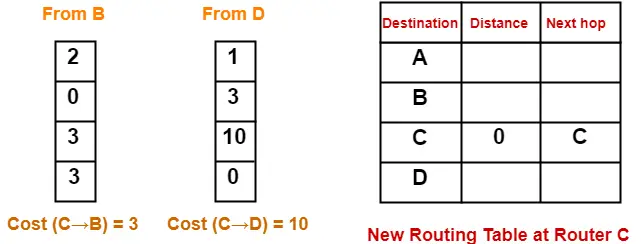• Cost of reaching destination A from router C = min { 3+2 , 10+1 } = 5 via B.
• Cost of reaching destination B from router C = min { 3+0 , 10+3 } = 3 via B.
• Cost of reaching destination D from router C = min { 3+3 , 10+0 } = 6 via B.

Thus, the new routing table at router C is-

 Destination Distance Next Hop A 5 B B 3 B C 0 C D 6 B

### At Router D-

• Router D receives distance vectors from its neighbors A, B and C.
• Router D prepares a new routing table as-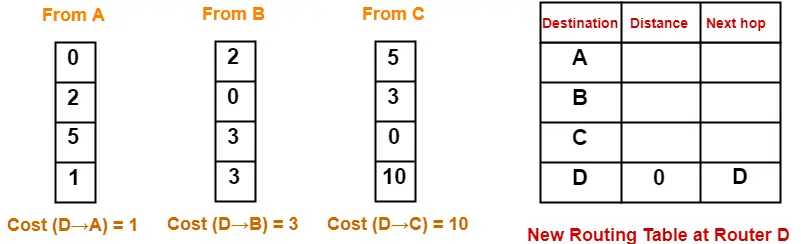• Cost of reaching destination A from router D = min { 1+0 , 3+2 , 10+5 } = 1 via A.
• Cost of reaching destination B from router D = min { 1+2 , 3+0 , 10+3 } = 3 via A.
• Cost of reaching destination C from router D = min { 1+5 , 3+3 , 10+0 } = 6 via A.

Thus, the new routing table at router D is-

 Destination Distance Next Hop A 1 A B 3 A C 6 A D 0 D

These will be the final routing tables at each router.

After routing tables converge (becomes stable),

• Some of the links connecting the routers may never be used.
• In the above example, we can identify the unused links as-

We have-

• The value of next hop in the final routing table of router A suggests that only edges AB and AD are used.
• The value of next hop in the final routing table of router B suggests that only edges BA and BC are used.
• The value of next hop in the final routing table of router C suggests that only edge CB is used.
• The value of next hop in the final routing table of router D suggests that only edge DA is used.

Thus, edges  BD and CD are never used.

## Note-01:

In Distance Vector Routing,

• Only distance vectors are exchanged.
• “Next hop”values are not exchanged.
• This is because it results in exchanging the large amount of data which consumes more bandwidth.

## Note-02:

While preparing a new routing table-

• A router takes into consideration only the distance vectors it has obtained from its neighboring routers.
• It does not take into consideration its old routing table.

## Note-03:

The algorithm is called so because-

• It involves exchanging of distance vectors between the routers.
• Distance vector is nothing but an array of distances.

## Note-04:

• The algorithm keeps on repeating periodically and never stops.
• This is to update the shortest path in case any link goes down or topology changes.

## Note-05:

• Routing tables are prepared total (n-1) times if there are n routers in the given network.
• This is because shortest path between any 2 nodes contains at most n-1 edges if there are n nodes in the graph.

## Note-06:

• Distance Vector Routing suffers from count to infinity problem.
• Distance Vector Routing uses UDP at transport layer.